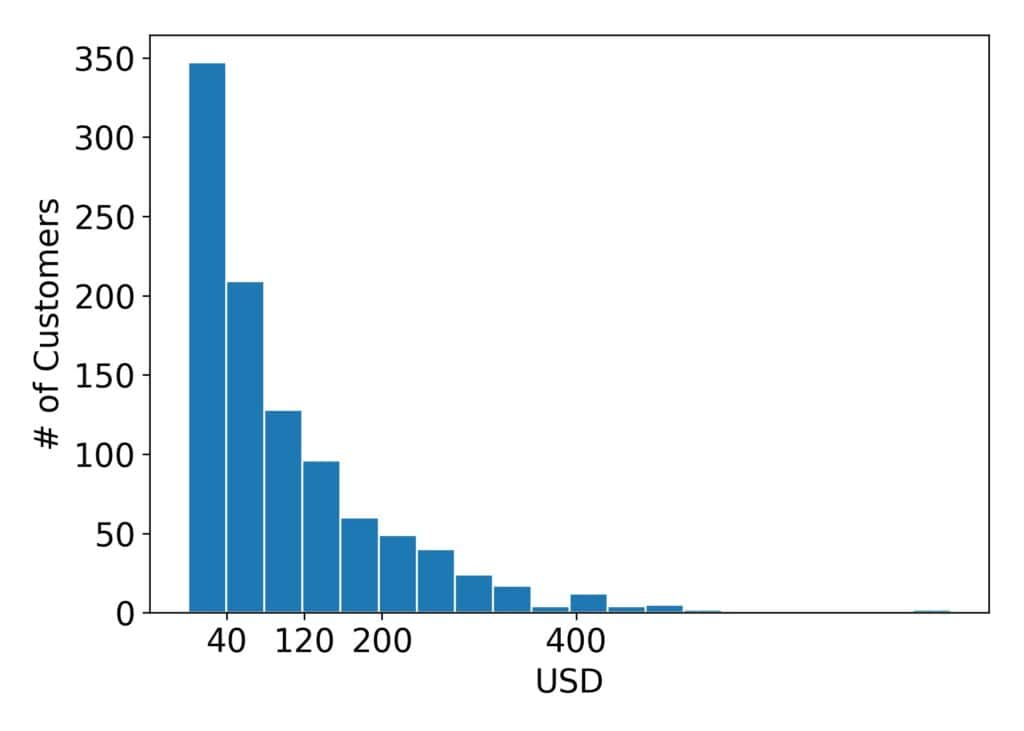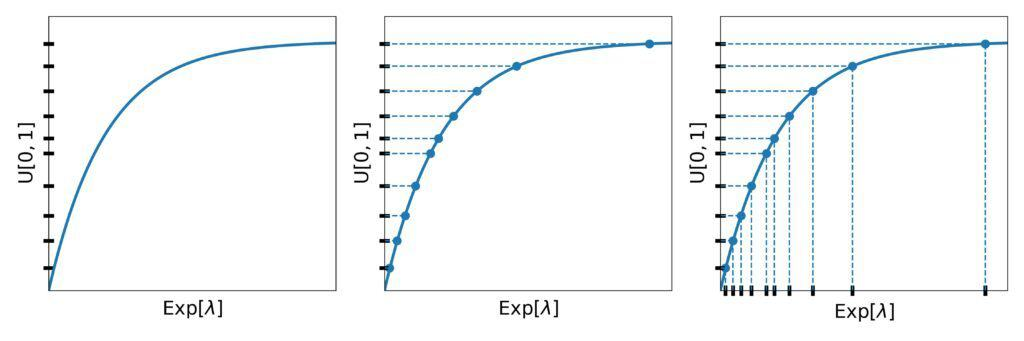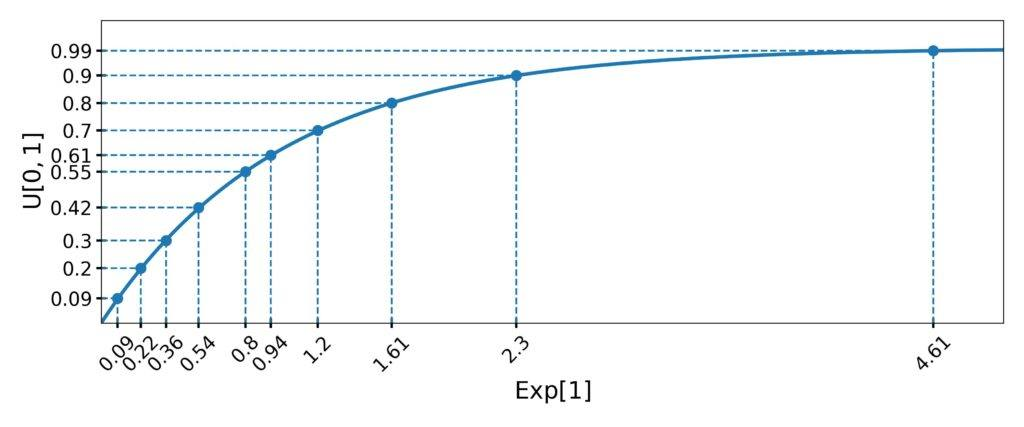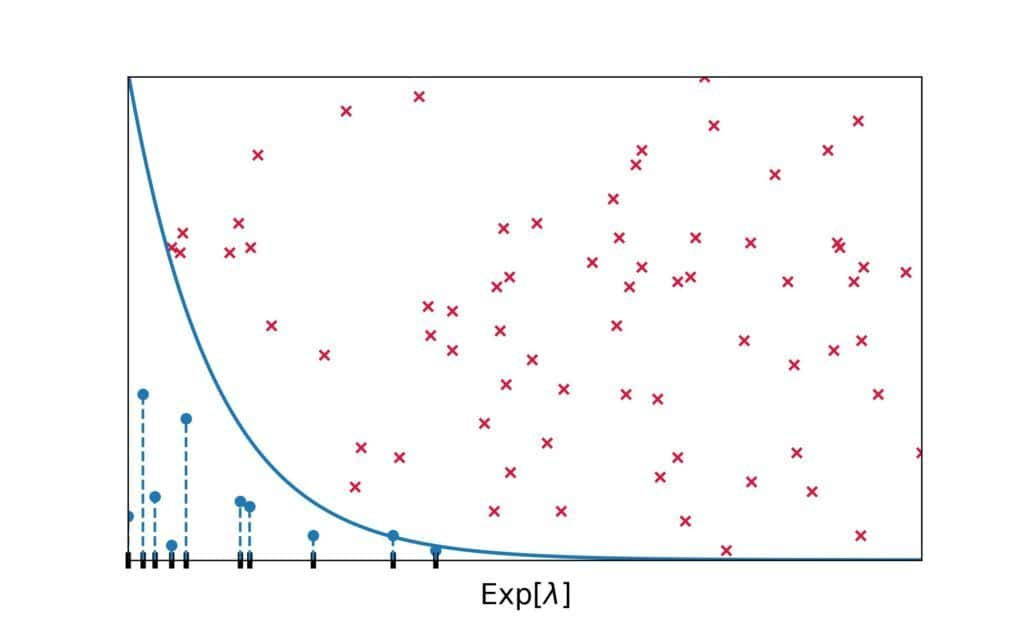## 1. Introduction

In this tutorial, we’ll implement a generator of pseudorandom numbers that follow an exponential distribution.

## 2. Exponential Distribution

Exponential distributions are useful for modeling the time passed until an event occurs. For instance, the duration of a call is distributed exponentially: short calls are frequent, whereas long ones are rare. Other phenomena also follow an exponential distribution. For instance, that’s the case with the amount of money customers spend on a trip to a market. Here’s an example of what a thousand customers typically spend in a supermarket:As we see, many customers spend USD 40 or less, but very few spend over USD 1000.

### 2.1. Math

An exponential distribution has a parameter. It quantifies the speed at which the occurrence probabilities of values decrease. The distribution’s probability density function (PDF) is:

(1)and its cumulative density function (CDF) is:

(2)The formulae show that the decrease speed (also known as decay) is exponential, hence the name.

## 3. The Inversion Method

The inversion method uses a property of CDFs: regarded as random variables, they follow the uniform distribution over:. What does that mean? Well, if we sample a lot of numbersfrom an exponential distribution and draw a histogram of the corresponding CDFs, we’ll see a uniform PDF.

But, the converse is also true. If we sampleuniform valuesfromand calculate the inverses, we’ll get numbers from the exponential distribution with the decay parameter,.

This method has a straightforward geometric interpretation. As the first step, we place thevalues on the-axis. Then, we find the points of theline whose-values are equal to the drawn. The found points’-values are the exponential numbers we wanted to draw:To use the method in programs, we need the inverseof the exponential CDF. We can derive it analytically:

(3)### 3.1. Pseudocode

Here’s the pseudocode of the inversion method:All we need isnumbers from, and the inversion method can transform them to followfor any.

Sincecomes from, the differencealso follows. So,  some implementations skip the subtraction step:

(4)### 3.2. Example

Let’s say we want ten numbers from.

First, we draw ten values from. For instance, let them be.

Then, we calculate the inverses:That way, we get ten numbers from:## 4. Rejection Sampling

To use the inversion method, we need the inverse. However, many distributions don’t have an invertible CDF, e.g., the normal distributions. Further, even if we can derive the inverse analytically, it may be too complex to compute efficiently, or we may not have an implementation in our software.

In such cases, we can apply rejection sampling. It uses the PDF plot of the target distribution and selects points from it randomly. If a selected point is below the PDF line, the method keeps its-coordinate. Otherwise, it rejects it. We repeat this step until collecting the desired number of samples.

Here’s the visual representation of rejection sampling for:Red crosses represent the rejected points, whereas blue circles denote those we kept. As we see, there are more accepted-values under the regions with higher overall densities.

Why does rejection sampling work? Since the PDF has high values in the high-density regions, they have a greater chance of capturing a randomly drawn point.

### 4.1. Pseudocode

Here’s the pseudocode of rejection sampling adapted to the exponential distribution:To select a random point in the plot according to a 2D uniform distribution, we can draw theandcoordinates independently.

Since the support ofis, we can propose any non-negative value as. However, using the maximal positive number as the bound would result in many rejected values and poor performance. So, we restrict sampling of the-coordinates to, whereis a suitable upper bound.

How do we choose? We can set it to a value that very rarely occurs. For instance,ofis to the left of. For, that is approximately 9.21.

Similarly, we samplefrom. That way, we speed up sampling because we won’t get points that are certainly above the PDF ofand have a zero chance of being kept.

Since the maximum density ofis atand is equal to, we sample-coordinates from.

### 4.2. Example

The first several iterations of rejection sampling for generating numbers fromcould go like this:Here, we can see a potential problem rejection sampling can face. It can generate many more points than we need. That can happen if the algorithm draws points mostly above the PDF line.

### 4.3. Optimization

One way to address this issue is to setto a smaller value. For instance, we can use the-quantile or-quantile of the target. However, trimming the distribution too much skews the generated numbers to the left. If that happened, we wouldn’t sample from, but from a similar yet different distribution.

We can also play with drawing points from the plot. Here, we used a 2D uniform distribution overto sample them, but any distribution would have worked. The only question is how to choose the one that best suits our target distribution.

## 5. Conclusion

In this article, we talked about sampling from an exponential distribution. We presented two techniques: the inversion method and rejection sampling.

The inversion method uses the inverse of the exponential CDF. Rejection sampling doesn’t require the inverse but can take longer.